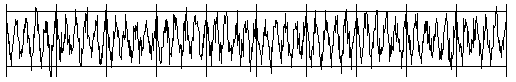deutsch     english    français     Print

## 8.6 CORRELATION, REGRESSION### INTRODUCTION

In both the natural sciences and in daily life, the collection of data in the form of measurements plays an important role. However, you do not always need to use a measuring instrument. The data can also consist in poll values, for example, in connection with a statistical investigation. After any data acquisition, it is most important to interpret the measured data. You may look for qualitative statements where the measured values rise or fall over time, or you might want experimentally verify a law of nature.

A major problem is that measurements are almost always subject to fluctuations and are not exactly reproducible. Despite these "measurement errors" people would still like to make scientifically correct statements. Measurement errors do not always emerge from flawed measuring instruments, but they may also lie in the nature of the experiment. For instance, the "measurement" of the numbers on a rolled die basically results in dispersed values between 1 and 6. Therefore, with any type of measurement, statistical considerations play a central role.

Often two variables x and y are measured together in a measurement series and questions arise as to whether these are related and if they are subject to regularity. Plotting the (x, y) values as measuring points in a coordinate system is called data visualization. You can almost always recognize whether the data are dependent of each other by simply looking at the distribution of the measured values.

 PROGRAMMING CONCEPTS: Data visualization, measured value distribution, cloud diagram, noise, covariance, correlation coefficient, regression, best fit

### VISUALIZING INDEPENDENT AND DEPENDENT DATA

 You can easily simulate independent data in a x-y diagram by using uniformly distributed random numbers for x and y. Although it is not necessary in this case, you copy the measured values into the data lists xval and yval and only then display them as data points.```from random import random
from gpanel import *

z = 10000

makeGPanel(-1, 11, -1, 11)
title("Uuniformly distributed value pairs")
drawGrid(10, 10, "gray")

xval =  * z
yval =  * z

for i in range(z):
xval[i] = 10 * random()
yval[i] = 10 * random()
move(xval[i], yval[i])
fillCircle(0.03)
```

Highlight program code (Ctrl+C to copy, Ctrl+V to paste)

 You get galaxy-like graphics if the x and y values are still independent of each other, but normally distributed around an average value. In this example, 5 is used as the average and 1 is used as the dispersion. This is also called a scatter plot or a cloud diagram. You can easily generate normally distributed random numbers by using random.gauss(average value, dispersion).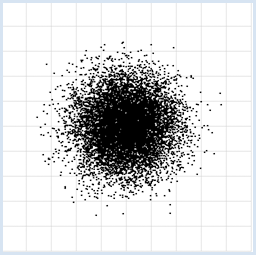```from random import gauss
from gpanel import *

z = 10000

makeGPanel(-1, 11, -1, 11)
title("Normally distributed value pairs")
drawGrid(10, 10, "gray")

xval =  * z
yval =  * z

for i in range(z):
xval[i] = gauss(5, 1)
yval[i] = gauss(5, 1)
move(xval[i], yval[i])
fillCircle(0.03)
```

Highlight program code (Ctrl+C to copy, Ctrl+V to paste)

 You can also simulate dependencies between x and y values by assuming that x increases in equidistant steps in a certain range, and y is a function of x that is, however, subject to statistical fluctuations. In physics, such fluctuations are often called noise. You draw the cloud diagram for a parabola y = -x * (0.2 * x - 2) and normally distributed noise in the area x = 0..10 with an increment size of 0.01.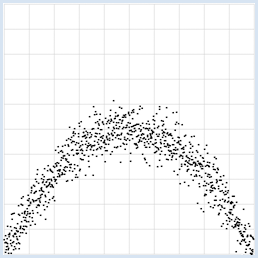```from random import gauss
from gpanel import *
import math

z = 1000
a = 0.2
b = 2

def f(x):
y = -x * (a * x - b)
return y

makeGPanel(-1, 11, -1, 11)
title("y = -x * (0.2 * x - 2) with normally distributed noise")
drawGrid(0, 10, 0, 10, "gray")

xval =  * z
yval =  * z

for i in range(z):
x = i / 100
xval[i] = x
yval[i] = f(x) + gauss(0, 0.5)
move(xval[i], yval[i])
fillCircle(0.03)
```

Highlight program code (Ctrl+C to copy, Ctrl+V to paste)

### MEMO

 You can recognize interdependencies of measured variables immediately through the use of data visualization [more... This example is characterized famous because correlation does not recognize the dependence]. With uniformly distributed random numbers in an interval [a, b], the numbers appear with the same frequency in each subinterval of equal length. Normally distributed numbers have a bell-shape distribution, where 68% of the numbers lie inside the interval (average - dispersion) and (average + dispersion).

### COVARIANCE AS A MEASURE FOR DEPENDENCY

 Here the goal is to not only make interdependencies visible in a diagram, but also to express them by a number. As often, you start from a concrete example and consider the double rolling of a die, where x is the number you get from the first roll and y is the number from the second roll. You conduct the rolling experiment many times and draw the value pairs as a cloud diagram. Since both measurement values of x and y are independent, you obtain a regular point cloud. If you calculate the expected value of x and y, it results in the generally known 3.5.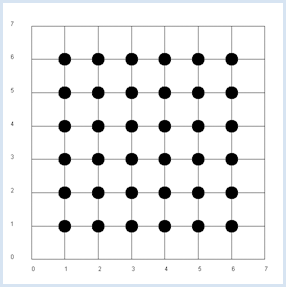Since x and y are independent, the probability pxy, to get the pair x, y from a double roll is pxy = px * py.

It is an obvious assumption that in the general case the following product rule applies.

If x and y are independent, the expected value of x * y equals the product of the expected values of x and y [more... The theoretical proof is simple: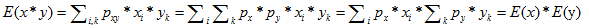] .

This assumption is confirmed in the simulation.

```from random import randint
from gpanel import *

z = 10000 # number of double rolls

def dec2(x):
return str(round(x, 2))

def mean(xval):
n = len(xval)
count = 0
for i in range(n):
count += xval[i]
return count / n

makeGPanel(-1, 8, -1, 8)
title("Double rolls. Independent random variables")
drawGrid(0, 7, 0, 7, 7, 7, "gray")

xval =   * z
yval =   * z
xyval =  * z

for i in range(z):
a = randint(1, 6)
b = randint(1, 6)
xval[i] = a
yval[i] = b
xyval[i] = xval[i] * yval[i]
move(xval[i], yval[i])
fillCircle(0.2)

xm = mean(xval)
ym = mean(yval)
xym = mean(xyval)
setStatusText("E(x) = " + dec2(xm) + \
",  E(y) = " + dec2(ym) + \
",  E(x, y) = " + dec2(xym))
```

Highlight program code (Ctrl+C to copy, Ctrl+V to paste)

### MEMO

 It is advisable to use a status bar where the results can be written out. The function dec2() rounds the value to 2 digits and returns it as a string. The values are of course subject to a statistical fluctuation. In the next simulation you will no longer analyze the rolled pair of numbers, but rather the numbers x of the first die and the sum y of the two rolled numbers. It is now evident that y is dependent on x, for example if x = 1, the probability of y = 4 is not the same as when x = 2.

 Your simulation confirms that the product rule indeed no longer applies if x and y are interdependent. It is therefore reasonable to introduce the deviation from the product rule, the difference c = E(x*y) - E(x)*E(y) called covariance, as a measure of the dependency between x and y. Simultaneously, you see in your program that the covariance can also be calculated as the sum of all square deviations from the average.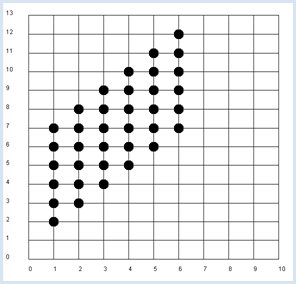```from random import randint
from gpanel import *

z = 10000 # number of double rolls

def dec2(x):
return str(round(x, 2))

def mean(xval):
n = len(xval)
count = 0
for i in range(n):
count += xval[i]
return count / n

def covariance(xval, yval):
n = len(xval)
xm = mean(xval)
ym = mean(yval)
cxy = 0
for i in range(n):
cxy += (xval[i] - xm) * (yval[i] - ym)
return cxy / n

makeGPanel(-1, 11, -2, 14)
title("Double rolls. Independent random variables")
drawGrid(0, 10, 0, 13, 10, 13, "gray")

xval =   * z
yval =   * z
xyval =  * z

for i in range(z):
a = randint(1, 6)
b = randint(1, 6)
xval[i] = a
yval[i] = a + b
xyval[i] = xval[i] * yval[i]
move(xval[i], yval[i])
fillCircle(0.2)

xm = mean(xval)
ym = mean(yval)
xym = mean(xyval)
c = xym - xm * ym
setStatusText("E(x) = " + dec2(xm) + \
",  E(y) = " + dec2(ym) + \
",  E(x, y) = " + dec2(xym) + \
",  c = " + dec2(c) + \
",  covariance = " + dec2(covariance(xval, yval)))
```

Highlight program code (Ctrl+C to copy, Ctrl+V to paste)

### MEMO

 You get a value of about 2.9 for the covariance in the simulation. The covariance is therefore well qualified as a measure for the dependence of random variables.

### THE CORRELATION COEFFICIENT

The just introduced covariance also has a disadvantage. Depending on how the measured values x and y are scaled, different values occur, even if the values are apparently equally dependent on each other. This is easy to see. If you take, for example, two dice that have sides with numbers going from 10 to 60 instead of from 1 to 6, the covariance changes greatly even though the dependence is the same. This is why you introduce a normalized covariance, called a correlation coefficient, by dividing the covariance by the dispersion of the x and y values

 correlation coefficient(x, y) = (         covariance(x, y)        )/ dispersion(x) * dispersion(y)

The correlation coefficient is always in the range from -1 to 1. A value close to 0 corresponds to a small dependence and a value close to 1 represents a large dependence, whereas increasing values of x correspond to increasing values of y, and a value close to -1 corresponds to increasing values of x and decreasing values of y.

You again use the double roll in the program and analyze the dependence of the sum of the numbers from the number of the first die.

```from random import randint
from gpanel import *
import math

z = 10000 # number of double rolls
k = 10 # scalefactor

def dec2(x):
return str(round(x, 2))

def mean(xval):
n = len(xval)
count = 0
for i in range(n):
count += xval[i]
return count / n

def covariance(xval, yval):
n = len(xval)
xm = mean(xval)
ym = mean(yval)
cxy = 0
for i in range(n):
cxy += (xval[i] - xm) * (yval[i] - ym)
return cxy / n

def deviation(xval):
n = len(xval)
xm = mean(xval)
sx = 0
for i in range(n):
sx += (xval[i] - xm) * (xval[i] - xm)
sx = math.sqrt(sx / n)
return sx

def correlation(xval, yval):
return covariance(xval, yval) / (deviation(xval) * deviation(yval))

makeGPanel(-1 * k, 11 * k, -2 * k, 14 * k)
title("Double rolls. Independent random variables.")
drawGrid(0, 10 * k, 0, 13 * k, 10, 13, "gray")

xval =   * z
yval =   * z
xyval =  * z

for i in range(z):
a = k * randint(1, 6)
b = k * randint(1, 6)
xval[i] = a
yval[i] = a + b
xyval[i] = xval[i] * yval[i]
move(xval[i], yval[i])
fillCircle(0.2 * k)

xm = mean(xval)
ym = mean(yval)
xym = mean(xyval)
c = xym - xm * ym
setStatusText("E(x) = " + dec2(xm) + \
",  E(y) = " + dec2(ym) + \
",  E(x, y) = " + dec2(xym) + \
",  covariance = " + dec2(covariance(xval, yval)) + \
",  correlation = " + dec2(correlation(xval, yval)))
```

Highlight program code (Ctrl+C to copy, Ctrl+V to paste)

### MEMO

 You can change the scaling factor k and the correlation will stay around 0.71, as opposed to the covariance which greatly changes [more... The correlation coefficient checks only linear dependencies. You turn it, for example, to the above parabolic cloud map, so there is a value close to 0, although the values associated obvious]. Instead of always calling it the correlation coefficient, you can simply call it correlation.

### MEDICAL RESEARCH PUBLICATION

 With the knowledge you have gained, you are already capable of understanding and evaluating a scientific publication, published in the year 2012 in the prestigious journal "New England Journal of Medicine" [more... FH Messerli, Chocolate Comsumption, Cognitive Function, and Nobel Laureates, The New England Journal of Medicine 367; 16 (Oct. 2012)]. The connection between consumers of chocolate and the number of Nobel Prize winners is investigated in different industrialized countries, or in other words, the question is considered whether there is a correlation between eating chocolate and intelligence. In the article the author uses the following data sources which you can also find on the Internet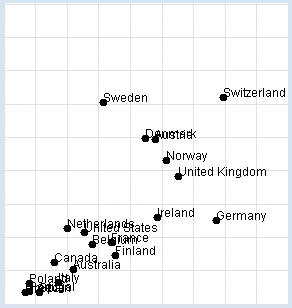Nobel Prizes:
http://en.wikipedia.org/wiki/List_of_countries_by_Nobel_laureates_per_capita

Chocolate consumption:
http://www.chocosuisse.ch/web/chocosuisse/en/documentation/facts_figures.html
http://www.theobroma-cacao.de/wissen/wirtschaft/international/konsum

You want to reconstruct the investigation yourself. In your program, you use a list data with sub-lists of three elements: the name of the country, the amount of chocolate consumption in kg per year and per inhabitant, and the number of Nobel Prize winners per 10 million inhabitants. You display the data graphically and determine the correlation coefficient.

```from gpanel import *
import math

data = [["Australia", 4.8, 5.141],
["Austria", 8.7, 24.720],
["Belgium", 5.7, 9.005],
["Denmark", 8.2, 24.915],
["Finland", 6.8, 7.371],
["France", 6.6, 9.177],
["Germany", 11.6, 12.572],
["Greece", 2.5, 1.797],
["Italy", 4.1, 3.279],
["Ireland", 8.8, 12.967],
["Netherlands", 4.5, 11.337],
["Norway", 9.2, 21.614],
["Poland", 2.7, 3.140],
["Portugal", 2.7, 1.885],
["Spain", 3.2, 1.705],
["Sweden", 6.2, 30.300],
["Switzerland", 11.9, 30.949],
["United Kingdom", 9.8, 19.165],
["United States", 5.3, 10.811]]

def dec2(x):
return str(round(x, 2))

def mean(xval):
n = len(xval)
count = 0
for i in range(n):
count += xval[i]
return count / n

def covariance(xval, yval):
n = len(xval)
xm = mean(xval)
ym = mean(yval)
cxy = 0
for i in range(n):
cxy += (xval[i] - xm) * (yval[i] - ym)
return cxy / n

def deviation(xval):
n = len(xval)
xm = mean(xval)
sx = 0
for i in range(n):
sx += (xval[i] - xm) * (xval[i] - xm)
sx = math.sqrt(sx / n)
return sx

def correlation(xval, yval):
return covariance(xval, yval) / (deviation(xval) * deviation(yval))

makeGPanel(-2, 17, -5, 55)
drawGrid(0, 15, 0, 50, "lightgray")

xval = []
yval = []

for country in data:
d = country
v = country
xval.append(d)
yval.append(v)
move(d, v)
fillCircle(0.2)
text(country)

title("Chocolate-Brainpower-Correlation: " + dec2(correlation(xval, yval)))
```

Highlight program code (Ctrl+C to copy, Ctrl+V to paste)

### MEMO

 This results in a high correlation of approximately 0.71, which can be interpreted in different ways. It is right to say that there is a correlation between the two sets of data, but we can only speculate about the reasons why. In particular a causal relationship between the consumption of chocolate and intelligence can by no means be proven this way. As a general rule: When there is a high correlation between x and y, x can be the cause for the behavior of y. Likewise, y can be the cause for the behavior of x, or x and y can be associated with other perhaps unknown causes. Discuss the meaning and purpose of this investigation with other people in your community and ask them for their opinion.

### MEASUREMENT ERRORS AND NOISE

 Even with an exact relationship between x and y, measurement errors or other influences can result in fluctuating measured values. In this simulation you assume a linear relationship between x and y, whereby the function values y are subjected to normally distributed fluctuations. The program calculates the correlation coefficient and draws the point with the coordinates (xm, ym) where xm and ym are the expected values of x and y, respectively.```from random import gauss
from gpanel import *
import math

z = 1000
a = 0.6
b = 2
sigma = 1

def f(x):
y = a * x + b
return y

def dec2(x):
return str(round(x, 2))

def mean(xval):
n = len(xval)
count = 0
for i in range(n):
count += xval[i]
return count / n

def covariance(xval, yval):
n = len(xval)
xm = mean(xval)
ym = mean(yval)
cxy = 0
for i in range(n):
cxy += (xval[i] - xm) * (yval[i] - ym)
return cxy / n

def deviation(xval):
n = len(xval)
xm = mean(xval)
sx = 0
for i in range(n):
sx += (xval[i] - xm) * (xval[i] - xm)
sx = math.sqrt(sx / n)
return sx

def correlation(xval, yval):
return covariance(xval, yval) / (deviation(xval) * deviation(yval))

makeGPanel(-1, 11, -1, 11)
title("y = 0.6 * x + 2 normally distributed measurement errors")
drawGrid(0, 10, 0, 10, "gray")
setColor("blue")
lineWidth(3)
line(0, f(0), 10, f(10))

xval =  * z
yval =  * z

setColor("black")
for i in range(z):
x = i / 100
xval[i] = x
yval[i] = f(x) + gauss(0, sigma)
move(xval[i], yval[i])
fillCircle(0.03)

xm = mean(xval)
ym = mean(yval)
move(xm, ym)
circle(0.5)

setStatusText("E(x) = " + dec2(xm) + \
",  E(y) = " + dec2(ym) + \
",  correlation = " + dec2(correlation(xval, yval)))
```

Highlight program code (Ctrl+C to copy, Ctrl+V to paste)

### MEMO

 This results in a high correlation as expected, which becomes even greater the smaller the selected dispersion sigma is. The correlation is exactly 1 for sigma = 0. Also, the point with the expected values P(0.5, 0.5) lies on the straight line.

### REGRESSION LINE, BEST FIT

Previously you started with a straight line, that you made "noisy" yourself. Here, you ask the opposite question: How do you detect the straight line again if you only have the two series of measurements? The desired line is called the regression line.

At least you already know that the regression line passes through the point P with the expected values. To find it, you can do the following:

 You place any line through P and determine the squares of the deviations from the measured values for all x from the straight line (vertical distance). The deviations must be as small as possible for the regression line. For this, you determine an error amount by adding all individual deviations together. You can find the best line in the simulation by gradually turning your placed line around the point P and always calculating the error sum, which will decline. You will have found the best fit just before the error sum begins to rise again.```from random import gauss
from gpanel import *
import math

z = 1000
a = 0.6
b = 2

def f(x):
y = a * x + b
return y

def dec2(x):
return str(round(x, 2))

def mean(xval):
n = len(xval)
count = 0
for i in range(n):
count += xval[i]
return count / n

def covariance(xval, yval):
n = len(xval)
xm = mean(xval)
ym = mean(yval)
cxy = 0
for i in range(n):
cxy += (xval[i] - xm) * (yval[i] - ym)
return cxy / n

def deviation(xval):
n = len(xval)
xm = mean(xval)
sx = 0
for i in range(n):
sx += (xval[i] - xm) * (xval[i] - xm)
sx = math.sqrt(sx / n)
return sx

sigma = 1

makeGPanel(-1, 11, -1, 11)
title("Simulate data points. Press a key...")
drawGrid(0, 10, 0, 10, "gray")
setStatusText("Press any key")

xval =  * z
yval =  * z

for i in range(z):
x = i / 100
xval[i] = x
yval[i] = f(x) + gauss(0, sigma)
move(xval[i], yval[i])
fillCircle(0.03)

getKeyWait()
xm = mean(xval)
ym = mean(yval)
move(xm, ym)
lineWidth(3)
circle(0.5)

def g(x):
y = m * (x - xm) + ym
return y

def errorSum():
count = 0
for i in range(z):
x = i / 100
count += (yval[i] - g(x)) * (yval[i] - g(x))
return count

m = 0
setColor("red")
lineWidth(1)
error_min = 0
while m < 5:
line(0, g(0), 10, g(10))
if m == 0:
error_min = errorSum()
else:
if errorSum() < error_min:
error_min = errorSum()
else:
break
m += 0.01

title("Regression line found")
setColor("blue")
lineWidth(3)
line(0, g(0), 10, g(10))
setStatusText("Found slope: " +  dec2(m) + \
", Theory: " + dec2(covariance(xval, yval)
/(deviation(xval) * deviation(xval))))
```

Highlight program code (Ctrl+C to copy, Ctrl+V to paste)

### MEMO

Instead of using a computer simulation to find the best fit, you can also directly calculate the slope of the regression line.

 m = (covariance(x, y))/ dispersion(x)2

Since the line passes through the point P with the expected values E(x) and E(y), it thus has the linear equation:

y - E(y) = m * (x - E(x))

### EXERCISES

1.

The well-known Engel's law of economics states that the actual amount of money spent on food rises in households with a rising income, yet the proportion of income spent on food decreases. Show the accuracy of this using the data material.

a. Visualize the relationship between income and total food expenditure (amount of money spent)

b. Visualize the relationship between income and relative food expenditure

c. Determine the correlation between income and absolute food expenditure

d. Determine the regression line between income and absolute food expenditure

Data:
 Monthly income 4000 4100 4200 4300 4400 4500 4600 4700 4800 4900 Expenditure% 64 63.25 62.55 61.9 61.3 60.75 60.25 59.79 59.37 58.99

 5000 5100 5200 5300 5400 5500 5600 5700 5800 5900 6000 58.65 58.35 58.08 57.84 57.63 57.45 57.3 57.17 57.06 56.97 56.9

2.

The currently generally accepted evolutionary history of the universe assumes that there was a Big Bang a long time ago and since then, the universe expands. The main question is when the Big Bang dates back to, or what is called the age of the universe. The astronomer Hubble published his world famous studies in 1929, where he found that there is a linear relationship between the distance d of the galaxies and their escape velocity v. The Hubble law is:

v = H * d

(where H is the Hubble constant)

You can comprehend the astrophysical thought process by starting with the following experimental data obtained from the Hubble Space Telescope:

 Galaxy Distance  [Mpc) Velocity [km/s] NGC0300 2 133 NGC095 9.16 664 NGC1326A 16.14 1794 NGC1365 17.95 1594 NGC1425 21.88 1473 NGC2403 3.22 278 NGC2541 11.22 714 NGC2090 11.75 882 NGC3031 3.63 80 NGC3198 13.8 772 NGC3351 10 642 NGC3368 10.52 768 NGC3621 6.64 609 NGC4321 15.21 1433 NGC4414 17.7 619 NGC4496A 14.86 1424 NGC4548 16.22 1384 NGC4535 15.78 1444 NGC4536 14.93 1423 NGC4639 21.98 1403 NGC4725 12.36 1103 IC4182 4.49 318 NGC5253 3.15 232 NGC7331 14.72 999

1 Megaparsec (Mpc) = 3.09 * 1019km

 a) Plot the data in a scatter plot. Copy it to the data list into your program. data = [ ["NGC0300", 2.00, 133], ["NGC095", 9.16, 664], ["NGC1326A", 16.14, 1794], ["NGC1365", 17.95, 1594], ["NGC1425", 21.88, 1473], ["NGC2403", 3.22, 278], ["NGC2541", 11.22, 714], ["NGC2090", 11.75, 882], ["NGC3031", 3.63, 80], ["NGC3198", 13.80, 772], ["NGC3351", 10.0, 642], ["NGC3368", 10.52, 768], ["NGC3621", 6.64, 609], ["NGC4321", 15.21, 1433], ["NGC4414", 17.70, 619], ["NGC4496A", 14.86, 1424], ["NGC4548", 16.22, 1384], ["NGC4535", 15.78, 1444], ["NGC4536", 14.93, 1423], ["NGC4639", 21.98, 1403], ["NGC4725", 12.36, 1103], ["IC4182", 4.49, 318], ["NGC5253", 3.15, 232], ["NGC7331", 14.72, 999]] from Freedman et al, The Astrophysical Journal, 553 (2001) b) Show that the values correlate well and determine the slope H of the regression line. c) If you assume that the velocity v of a certain galaxy remains constant, its distance is d = v * T, where T is the age of the universe. Following the Hubble law (v = H * d) we can deduce T = 1 / H . Determine T.
.

### FINDING CACHED INFORMATION WITH AUTOCORRELATION

Intelligent beings on a distant planet want to contact other living beings. To do this, they send out radio signals that present a certain regularity (you can imagine a kind of Morse code). The signal becomes weaker and weaker on the long transmission path and gets masked by statistically fluctuating noise. We receive this signal on Earth with a radio telescope, and so for the time being we only hear the noise.

The statistics and a computer program can help us retrieve the original radio signal again. If you calculate the correlation coefficient of the signal with its own time-shifted signal, you decrease the statistical noise components. (A correlation with itself is called an autocorrelation).

 You can simulate this property important for signal analysis with a Python program. The original useful signal is a sine wave and you superimpose it on the noise by adding a random number to each sample value (with a normal distribution). Show the noisy signal in the upper part of the graph and wait for a key press. The useful signal is no longer recognizable. Subsequently, you build the autocorrelation of the signal and draw the course of the correlation coefficient in the lower part of the graph. The useful signal will be clearly recognizable again.```from random import gauss
from gpanel import *
import math

def mean(xval):
n = len(xval)
count = 0
for i in range(n):
count += xval[i]
return count / n

def covariance(xval, yval):
n = len(xval)
xm = mean(xval)
ym = mean(yval)
cxy = 0
for i in range(n):
cxy += (xval[i] - xm) * (yval[i] - ym)
return cxy / n

def deviation(xval):
n = len(xval)
xm = mean(xval)
sx = 0
for i in range(n):
sx += (xval[i] - xm) * (xval[i] - xm)
sx = math.sqrt(sx / n)
return sx

def correlation(xval, yval):
return covariance(xval, yval)/(deviation(xval)*deviation(yval))
def shift(offset):
signal1 =  * 1000
for i in range(1000):
signal1[i] = signal[(i + offset) % 1000]
return signal1

makeGPanel(-10, 110, -2.4, 2.4)
title("Noisy signal. Press a key...")
drawGrid(0, 100, -2, 2.0, "lightgray")

t = 0
dt = 0.1
signal =  * 1000
while t < 100:
y = 0.1 * math.sin(t) # Pure signal
#    noise = 0
noise = gauss(0, 0.2)
z = y + noise
if t == 0:
move(t, z + 1)
else:
draw(t, z + 1)
signal[int(10 * t)] = z
t += dt

getKeyWait()
title("Signal after autocorrelation")
for di in range(1, 1000):
y = correlation(signal, shift(di))
if di == 1:
move(di / 10, y - 1)
else:
draw(di / 10, y - 1)
```

Highlight program code (Ctrl+C to copy, Ctrl+V to paste)

To make it a bit more exciting, listen to the noisy signal first and then the extracted useful signal. To do this, tap into your knowledge from the chapter on Sound.

```from soundsystem import *
import math
from random import gauss
from gpanel import *

n = 5000

def mean(xval):
count = 0
for i in range(n):
count += xval[i]
return count / n

def covariance(xval, k):
cxy = 0
for i in range(n):
cxy += (xval[i] - xm) * (xval[(i + k) % n] - xm)
return cxy / n

def deviation(xval):
xm = mean(xval)
sx = 0
for i in range(n):
sx += (xval[i] - xm) * (xval[i] - xm)
sx = math.sqrt(sx / n)
return sx

makeGPanel(-100, 1100, -11000, 11000)
drawGrid(0, 1000, -10000, 10000)
title("Press <SPACE> to repeat. Any other key to continue.")

signal = []
for i in range(5000):
value = int(200 *  (math.sin(6.28 / 20 * i) + gauss(0, 4)))
signal.append(value)
if i == 0:
move(i, value + 5000)
elif i <= 1000:
draw(i, value + 5000)

ch = 32
while ch == 32:
openMonoPlayer(signal, 5000)
play()
ch = getKeyCodeWait()

signal1 = []
xm = mean(signal)
sigma = deviation(signal)
q = 20000 / (sigma * sigma)
for di in range(1, 5000):
value = int(q *  covariance(signal, di))
signal1.append(value)
title("Autocorrelation Done. Press any key to repeat.")
for i in range(1, 1000):
if i == 1:
move(i, signal1[i] - 5000)
else:
draw(i, signal1[i] - 5000)

while True:
openMonoPlayer(signal1, 5000)
play()
getKeyCodeWait()
```

Highlight program code (Ctrl+C to copy, Ctrl+V to paste)

### MEMO

 Instead of executing the program, you can listen to the two signals as WAV files here Noisy signal (click here)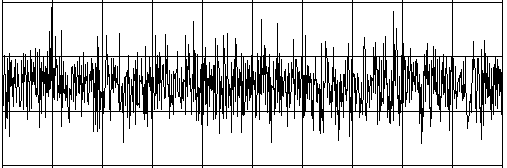Desired signal (click here)